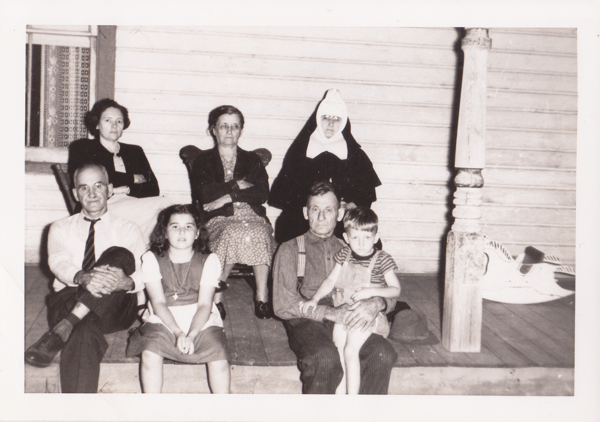## Front Porch

Place mouse over face for identification of personDescription Front Porch Date / Time 1947-07-00 Country / State / County / City / Placename CAN, ON, xx, Tweed Persons (t-b,l-r) Florence Agnes Goulah, Harriet (nee Goulah) Courneya, Sister (nee Amelia Goulah) Petronela, Noah Louis Goulah, Nannette Marie Goulah, Joseph Goulah
```lib card:
dc:
title 		=	Front Porch
creator 	=	Jerome Goulet
subject 	=
description 	=	Original (scanned analog or digital), names to faces, stored to LibCard "format", within image exif:
publisher 	=	Jerome Goulet
contributor 	=
date 		=	1947-07-00
resourcetype 	=
iformat 	=
if_mime 	=	image/png
if_resolution 	=	600x600
if_dimensions 	=
if_dim_x 	=	600
if_dim_y 	=	422
resourceID 	=
source 		=	photograph (analog or digital)
language 	=	eng
lc:
when:
dateUTC		=	1947-07-00
timeUTC		=	0000
tz		=	+0000

dateLocal	=	1947-07-00
timeLocal	=	0000
where:
pName		=	x
lat		=	x
lon		=	x
who:
person:
name:
surName		=	Goulah
givenName	=	Florence Agnes
aka		=	FAG1930
relation	=
pip:	(person in picture)
boxX		=	96
boxY		=	108
boxWidth	=	35
boxHeight	=	35
label		=
uri		=
person:
name:
surName		=	Courneya
givenName	=	Harriet (nee Goulah)
aka		=	HGC
relation	=
pip:	(person in picture)
boxX		=	211
boxY		=	113
boxWidth	=	37
boxHeight	=	37
label		=
uri		=
person:
name:
surName		=	Petronela
givenName	=	Sister (nee Amelia Goulah)
aka		=	AGP
relation	=
pip:	(person in picture)
boxX		=	318
boxY		=	113
boxWidth	=	37
boxHeight	=	37
label		=
uri		=
person:
name:
surName		=	Goulah
givenName	=	Noah Louis
aka		=	NLG1893
relation	=
pip:	(person in picture)
boxX		=	70
boxY		=	171
boxWidth	=	42
boxHeight	=	42
label		=
uri		=
person:
name:
surName		=	Goulah
givenName	=	Nannette Marie
aka		=	NMG1937
relation	=
pip:	(person in picture)
boxX		=	164
boxY		=	213
boxWidth	=	37
boxHeight	=	37
label		=
uri		=
person:
name:
surName		=	Goulah
givenName	=	Joseph
aka		=	JG
relation	=
pip:	(person in picture)
boxX		=	303
boxY		=	194
boxWidth	=	40
boxHeight	=	40
label		=
uri		=
what:
eventName	=	Front Porch
```